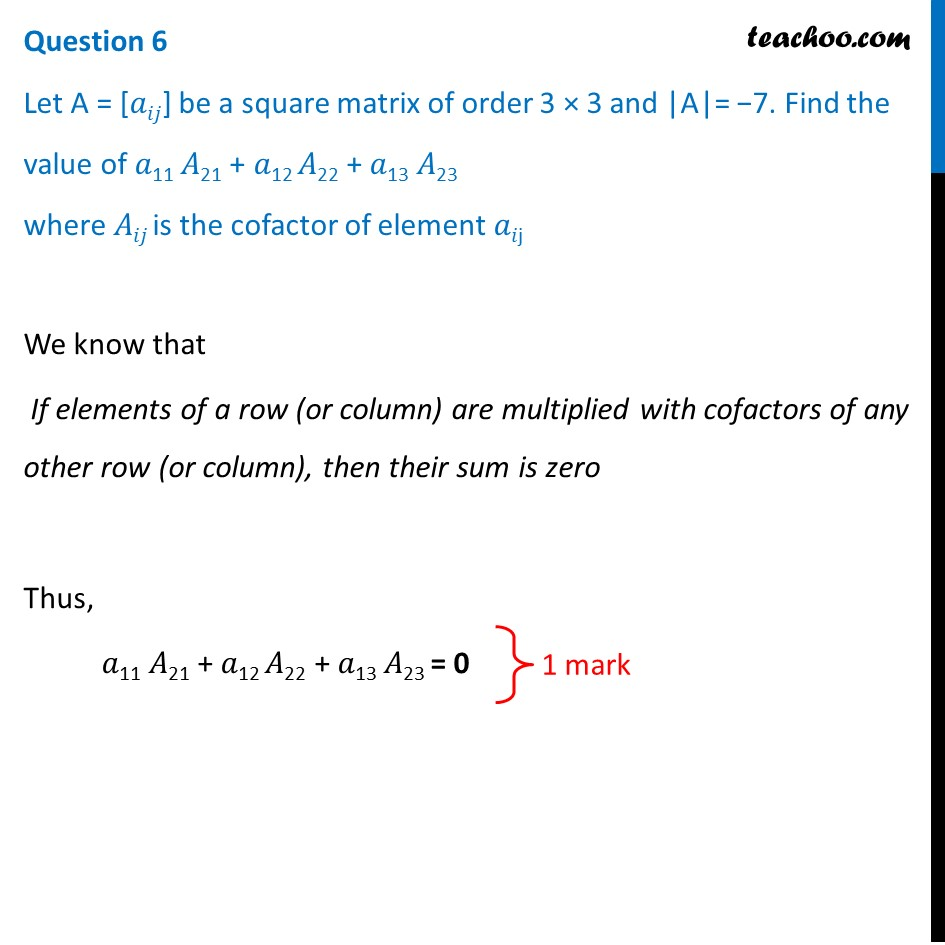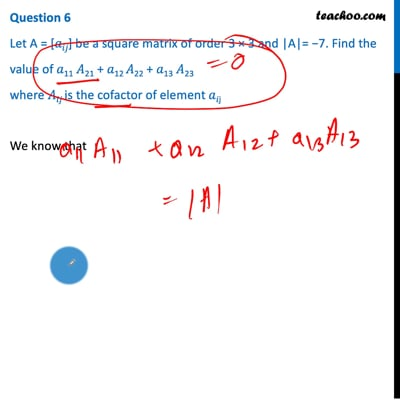CBSE Class 12 Sample Paper for 2021 Boards

Class 12
Solutions of Sample Papers and Past Year Papers - for Class 12 Boards

## Let A = [𝑎𝑖𝑗] be a square matrix of order 3×3 and |A|= -7. Find the value of 𝑎 11 𝐴 21 + 𝑎 12 𝐴 22 + 𝑎 13 𝐴 23  where 𝐴 𝑖𝑗 is the cofactor of element 𝑎 𝑖jThis video is only available for Teachoo black users

Introducing your new favourite teacher - Teachoo Black, at only ₹83 per month

### Transcript

Question 6 Let A = [𝑎𝑖𝑗] be a square matrix of order 3 × 3 and |A|= −7. Find the value of 𝑎11 𝐴21 + 𝑎12 𝐴22 + 𝑎13 𝐴23 where 𝐴𝑖𝑗 is the cofactor of element 𝑎𝑖j We know that ﻿If elements of a row (or column) are multiplied with cofactors of any other row (or column), then their sum is zero Thus, 𝑎11 𝐴21 + 𝑎12 𝐴22 + 𝑎13 𝐴23 = 0